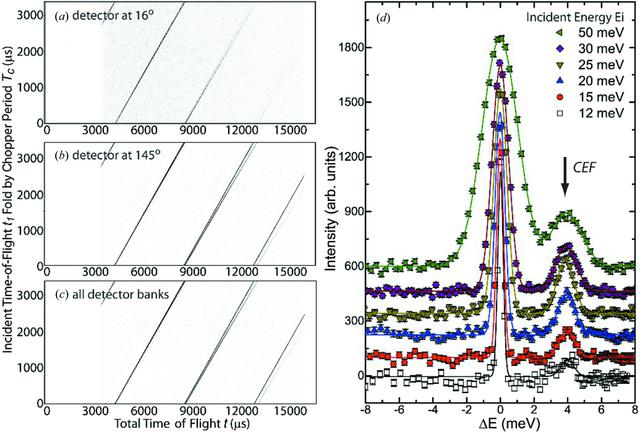disable zoom     view article Figure 2 (a)–(c) The reconstructed scattering intensity [proportional to S(q, ω)] for data collected at scattering angles 2θ = 16 and 145°, and summation over all detector banks, showing the coexistence of strong elastic and weak inelastic lines. The correlation chopper is running at a frequency f = 293.4118 Hz (periodicity TC = 3408 µs). The neutron events collected at the detector have a periodicity of (22.5/18)TC = 4260 µs, where 22.5 is the distance from the moderator to the detector (in metres) and 18 is the distance from the moderator to the correlation chopper (in metres). The incident time of flight t1 [y axis in panels (a)–(c)] has been folded by the chopper period TC, leaving four repetition windows in the plot. Note that the elastic line goes through the origin (t1 = 0, t = 0). The data were from a PrAl3 powder sample (mass of 4 g) at 5 K. (d) Horizontal line cuts performed for the data in panel (c), with the x-axis units converted to energy transfer (ΔE), i.e. the line cuts in panel (d) with Ei = 12 and 50 meV are converted from the data in panel (c) near total time of flight t = 14 851 and 7275 µs, respectively. The CEF excitation at ΔE = 4 meV of PrAl3 is clearly resolved for all incident neutron energies between 12 and 50 meV. For clarity, the data at 15, 20, 25, 30 and 50 meV have been shifted vertically by 120, 240, 360, 480 and 600, respectively.JOURNAL OFAPPLIEDCRYSTALLOGRAPHY
ISSN: 1600-5767
Volume 51| Part 2| April 2018| Pages 315-322